# DeMystifying Dynamic Systems Solution of Homogeneous ODE No

• Slides: 81
Download presentationDe-Mystifying Dynamic Systems • Solution of Homogeneous ODE • No Forcing Function • Only Complimentary Part • Exponential Solution • For Non-Homogeneous ODE • Forcing Function • Complimentary Part + Particular Integral • Exponential Solution + Soln. based on type of forcing fn.Control Engineering Philosophy Poles & Zeros ◦ Consider a Proper, Rational Polynomial Fraction ◦ Laplace Transform of a ODE/PDE or Transfer Function ◦ Roots of the Numerator are “Zeros” Drives the dynamics to zero or “rest” ◦ Roots of the Denominator are “Poles” Drives the dynamics to infinity or “unstable” regions Beginning of Control Engineering ◦ Engineering of Transients & DisturbancesControlling Dynamic Systems System ◦ ◦ ◦ Engineering Requirements Process, Equipment, Instrumentation, etc. Quick Response to Disturbances No Overloading Quick Settling Down Error Free Steady State Operation Control Engineers Job ◦ Transient State Response Time, Peak-Overshoot & Settling Time ◦ Steady State No Offsets ---- Integral ControlAdvances in Control Theory --- The State Space Framework Any Dynamic System comprises of particles termed as States No. of States, Same as Order of the System --- effectively the Number of Energy Storing Elements in the System Knowledge of the Position & Dynamics of the States, may Completely define the System Dynamics The Abstract Boundary of the Dynamic System States x x x Similar to Molecules in Newtonian Mechanics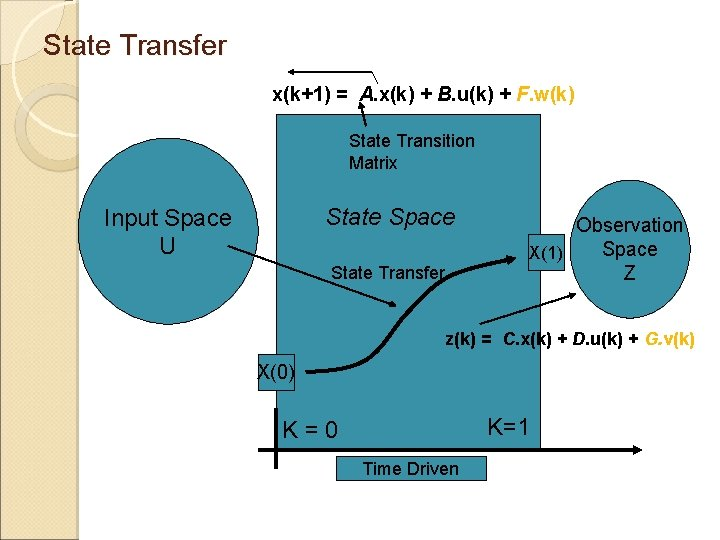State Transfer x(k+1) = A. x(k) + B. u(k) + F. w(k) State Transition Matrix State Space Input Space U State Transfer Observation Space X(1) Z z(k) = C. x(k) + D. u(k) + G. v(k) X(0) K=1 K=0 Time DrivenSystem Dynamics with Forcing Function & Noise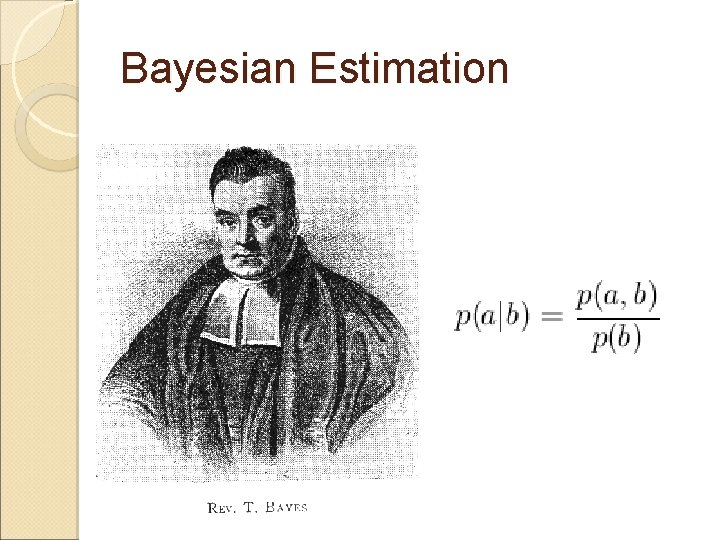Bayesian Estimation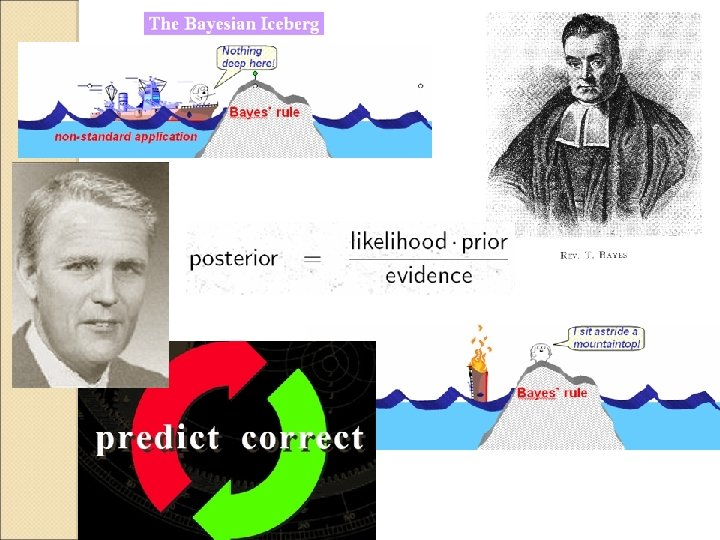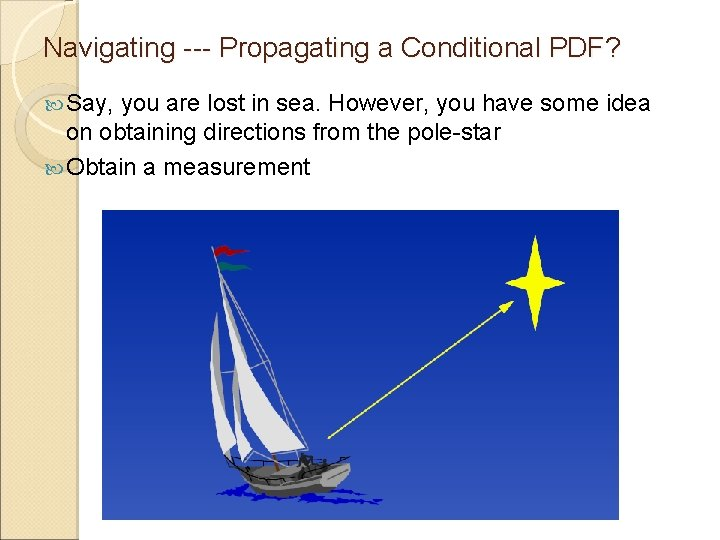Navigating --- Propagating a Conditional PDF? Say, you are lost in sea. However, you have some idea on obtaining directions from the pole-star Obtain a measurementAsess the Measurement The It measurement may not be exact would have a mean and a varianceUpdate the Previous Measurement Now, let’s say your friend is better in navigating He takes a second measurement --- more accurate ? Even this would have a mean and variance, but possibly the variance would be smaller – he has more expertise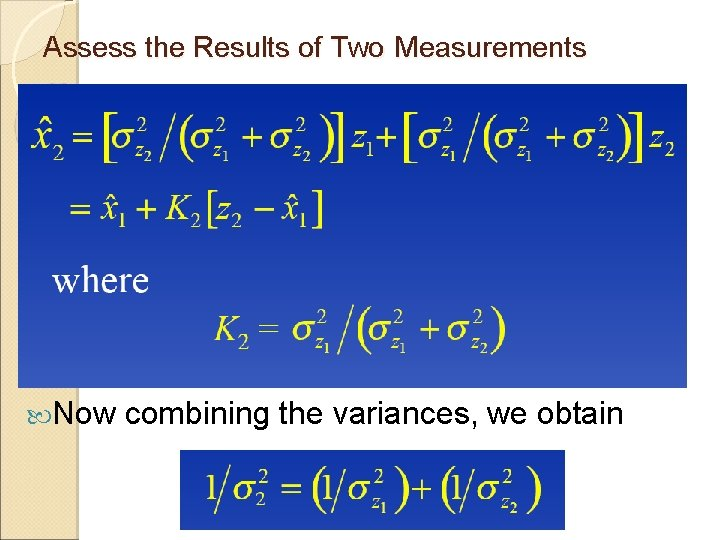Assess the Results of Two Measurements Now combining the variances, we obtainResult The result is an improved idea as to how much away you are from the coast Are we propagating a conditional density function and each time updating it with the latest measurement ?Adding Dynamics of the Boat, dx/dt = v + w Uncertainties (w)--- tide-drag, wind-speed, etc. Velocity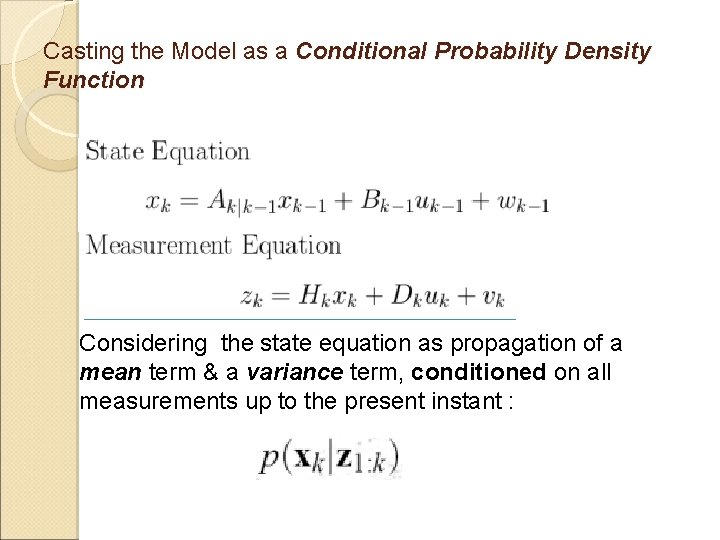Casting the Model as a Conditional Probability Density Function Considering the state equation as propagation of a mean term & a variance term, conditioned on all measurements up to the present instant :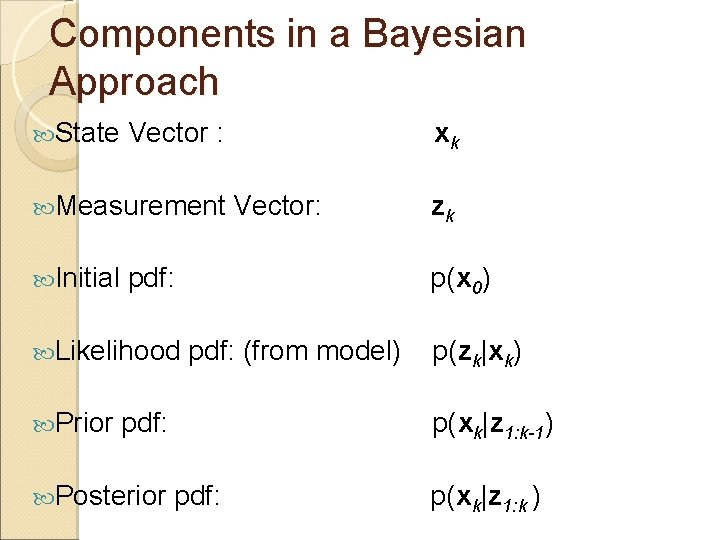Components in a Bayesian Approach State Vector : Measurement Initial Vector: pdf: (from model) pdf: Posterior zk p(x 0) Likelihood Prior xk p(zk|xk) p(xk|z 1: k-1) pdf: p(xk|z 1: k )Propagating from (k-1) to (k+1)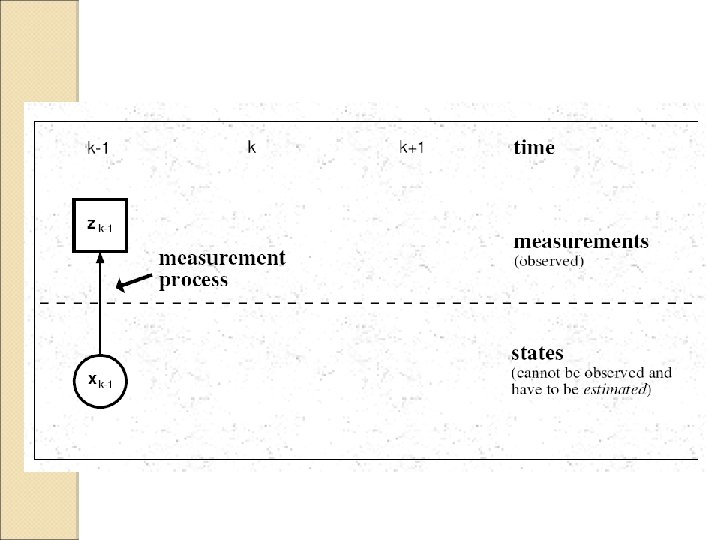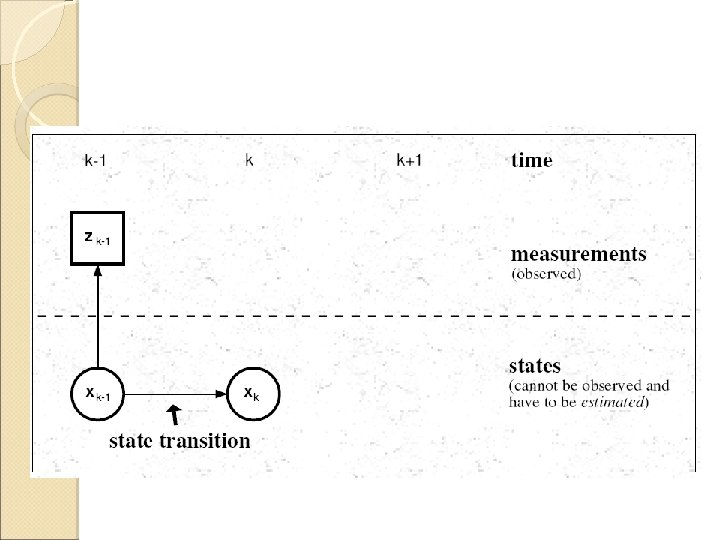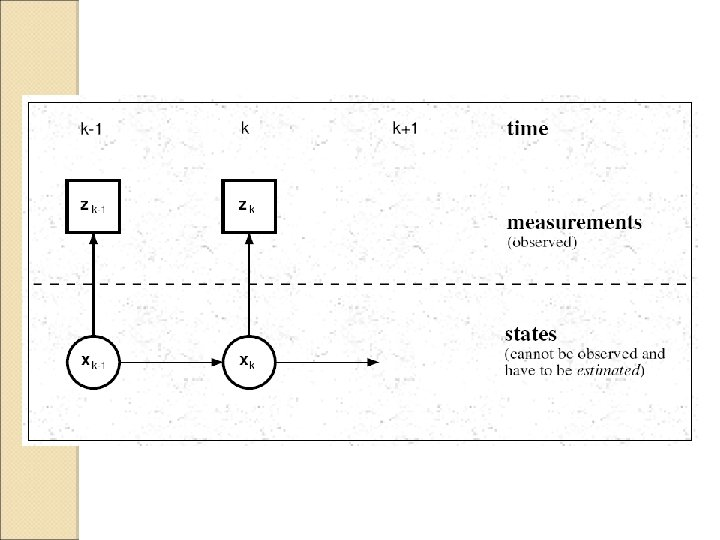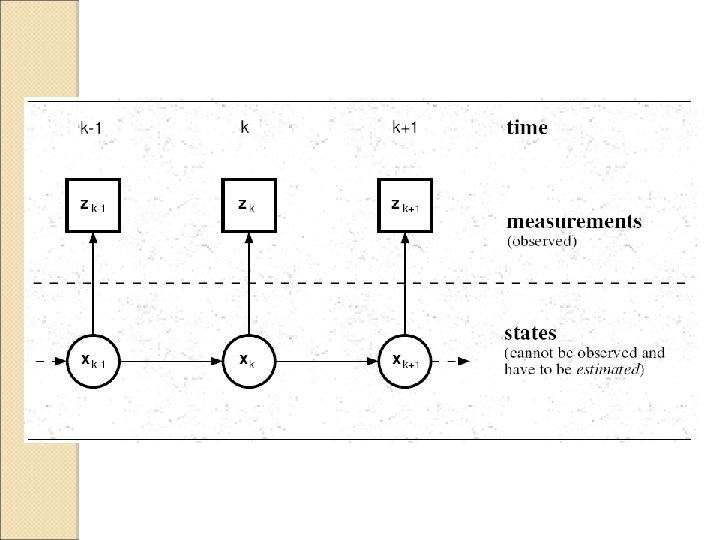State Transfer x(k+1) = A. x(k) + B. u(k) + F. w(k) State Transition Matrix State Space Input Space U State Transfer Observation Space X(1) Z z(k) = C. x(k) + D. u(k) + G. v(k) X(0) K=1 K=0 Time Driven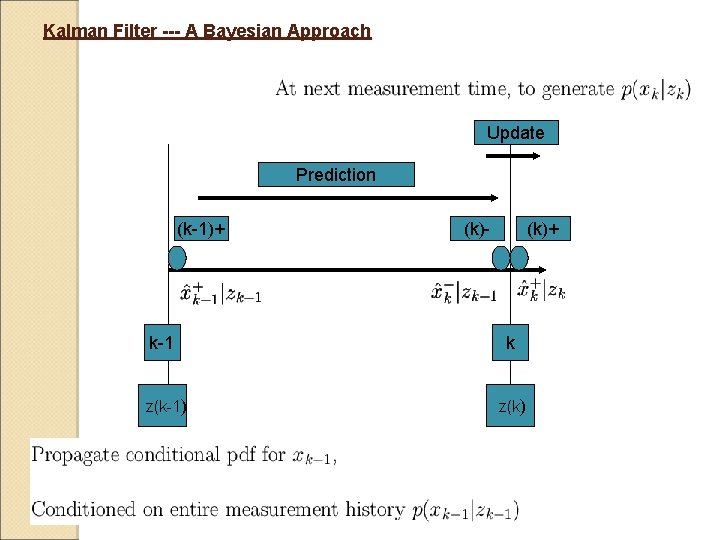Kalman Filter --- A Bayesian Approach Update Prediction (k-1)+ (k)- (k)+ k-1 k z(k-1) z(k)Structure of the Update EquationApplication of Baye’s Rule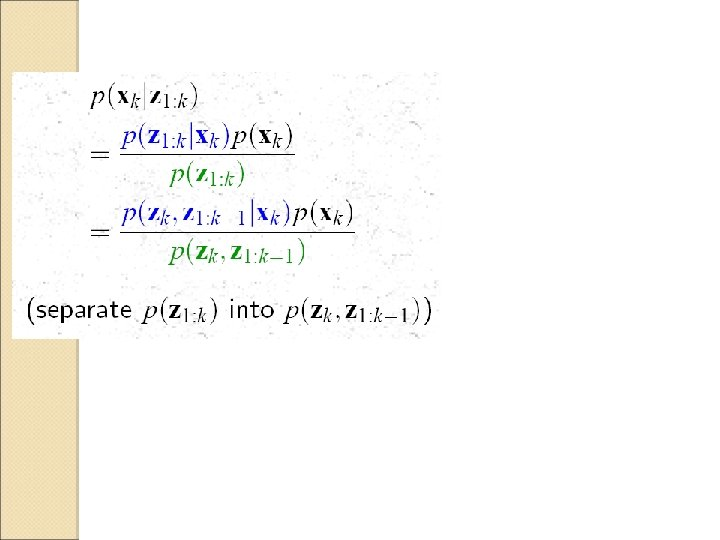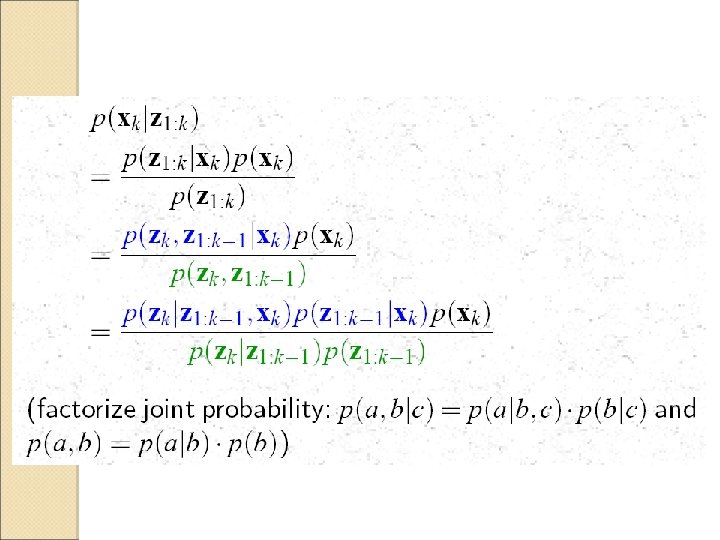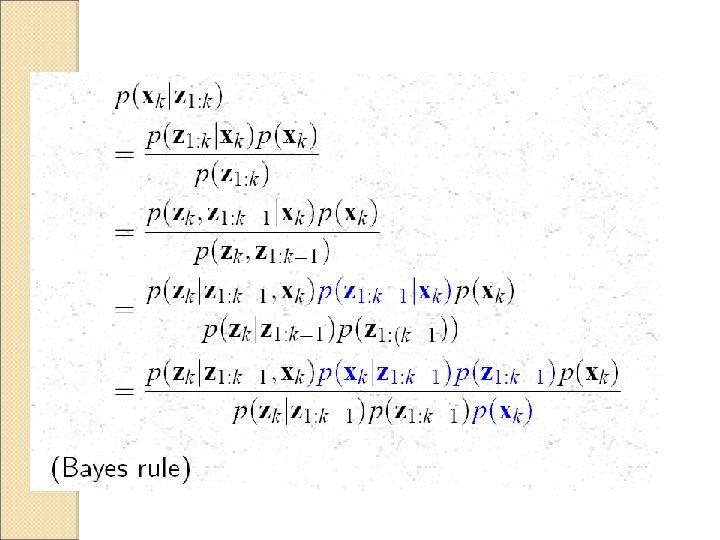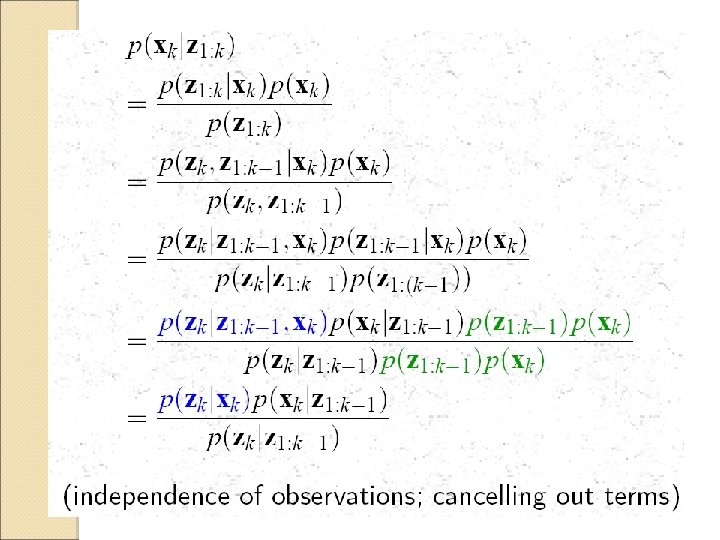Structure of the Update Equation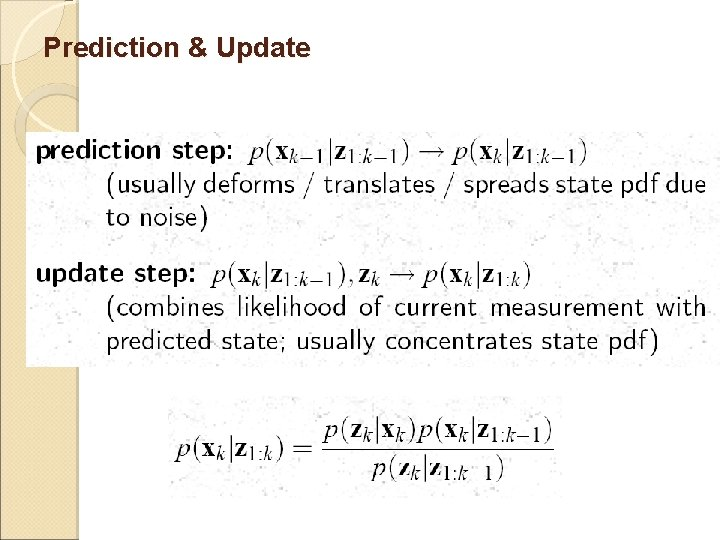Prediction & UpdateUpdate (k-1)+ (k)- (k)+ Prediction k-1 k z(k-1) z(k)Update (k-1)+ (k)- (k)+ Prediction k-1 k z(k-1) z(k)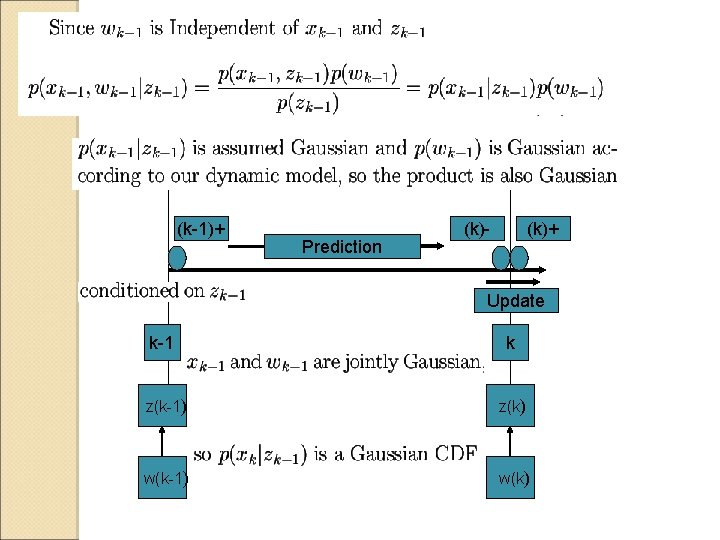(k-1)+ Prediction (k)- (k)+ Update k-1 k z(k-1) z(k) w(k-1) w(k)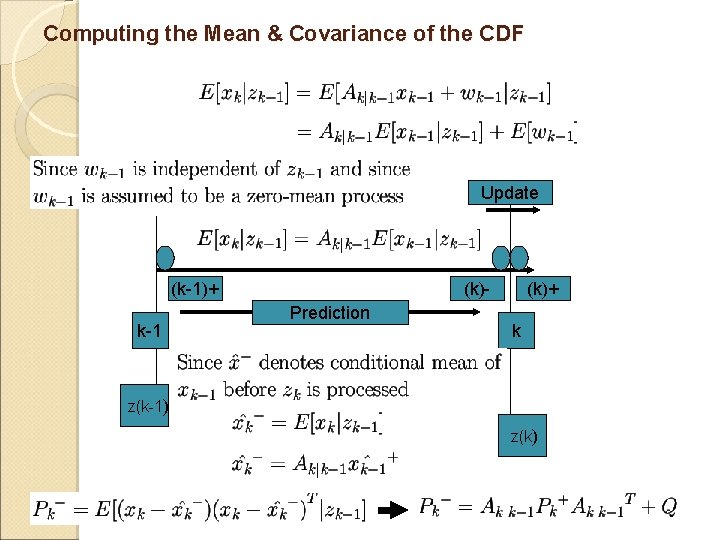Computing the Mean & Covariance of the CDF Update (k-1)+ k-1 (k)Prediction (k)+ k z(k-1) z(k)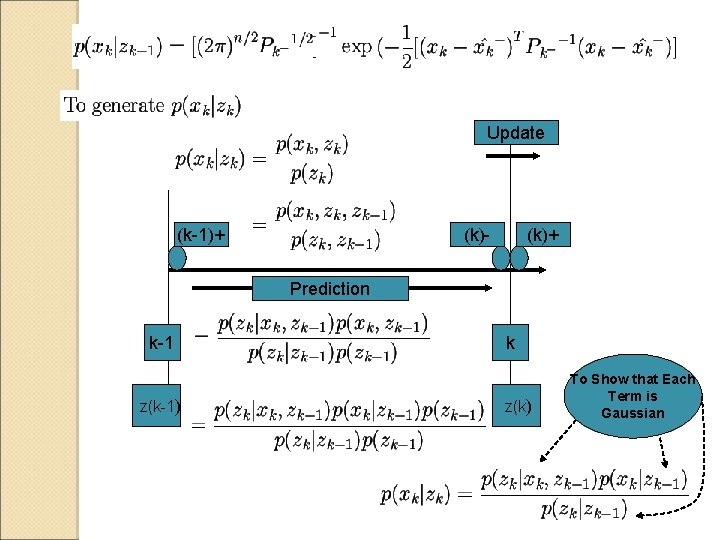Update (k-1)+ (k)- (k)+ Prediction k-1 z(k-1) k z(k) To Show that Each Term is Gaussian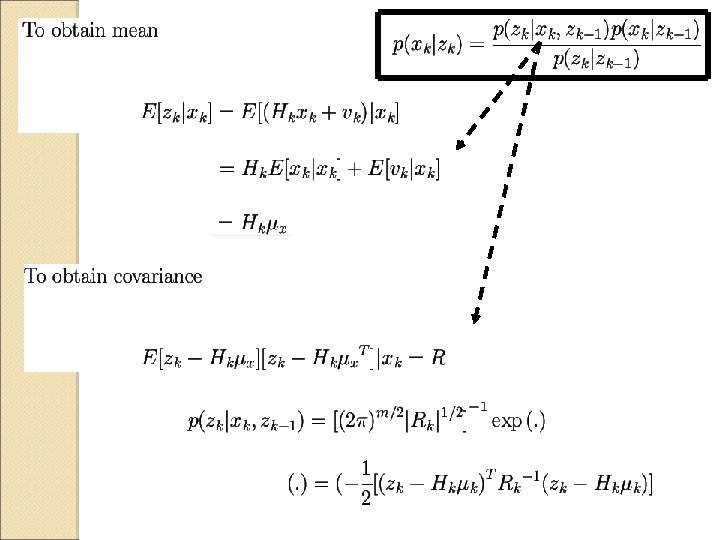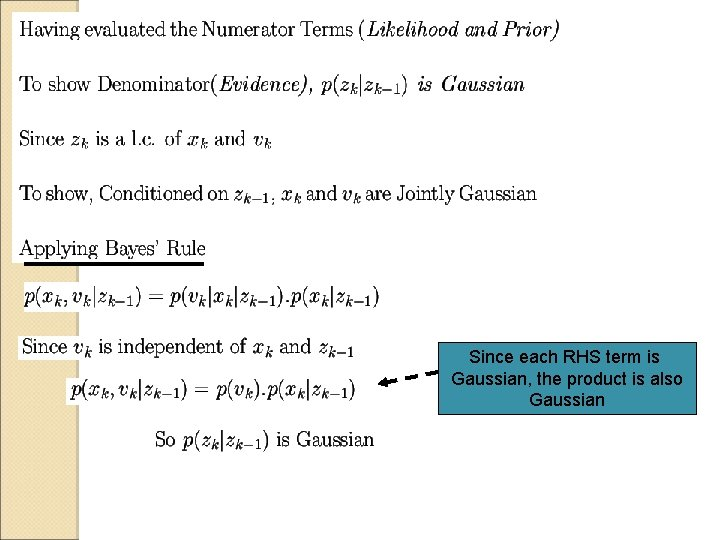Since each RHS term is Gaussian, the product is also Gaussian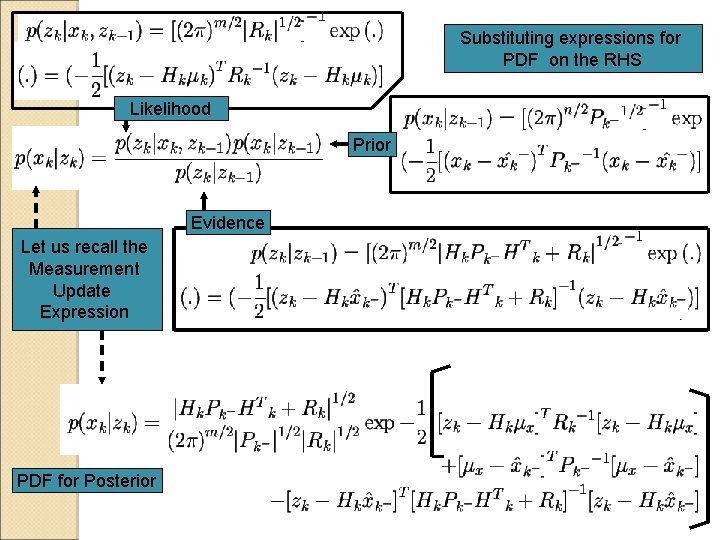Substituting expressions for PDF on the RHS Likelihood Prior Evidence Let us recall the Measurement Update Expression PDF for Posterior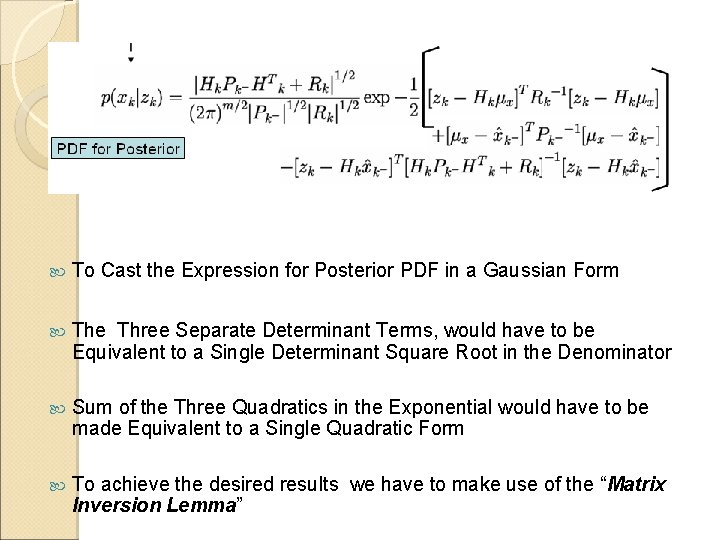To Cast the Expression for Posterior PDF in a Gaussian Form The Three Separate Determinant Terms, would have to be Equivalent to a Single Determinant Square Root in the Denominator Sum of the Three Quadratics in the Exponential would have to be made Equivalent to a Single Quadratic Form To achieve the desired results we have to make use of the “Matrix Inversion Lemma”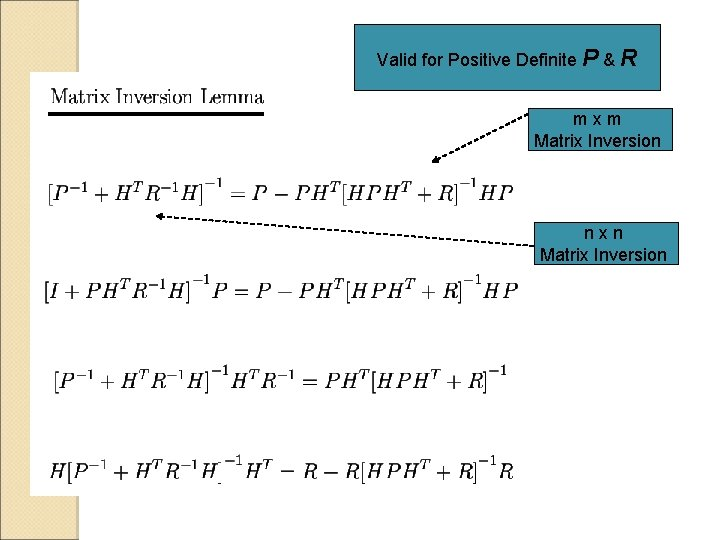Valid for Positive Definite P & R mxm Matrix Inversion nxn Matrix Inversion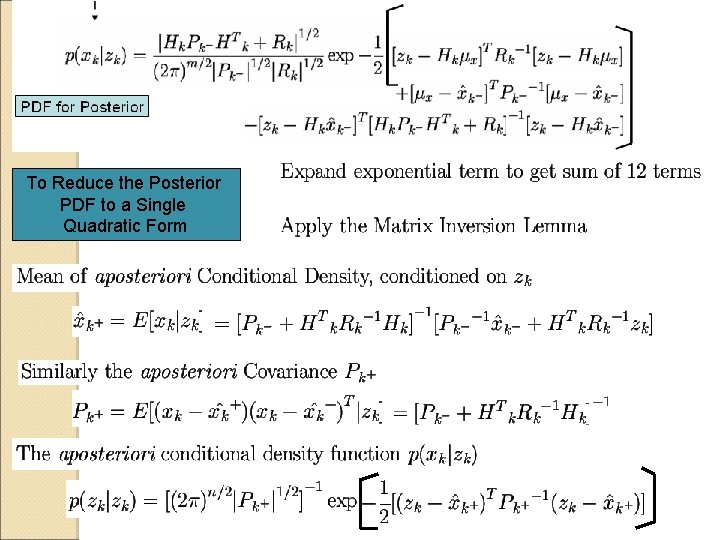To Reduce the Posterior PDF to a Single Quadratic FormFurther Application of the Matrix Inversion Lemma Casting in the earlier Condn. Mean Eqn. We obtain more efficient expressions for State & Covariance Using a term called the Kalman Gain The expressions for Condn. Mean & Covariance becomes Kalman Filter Update Equations ?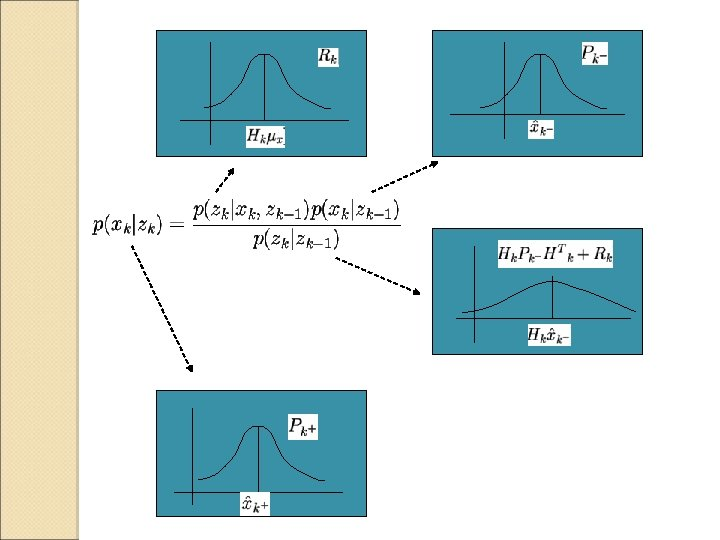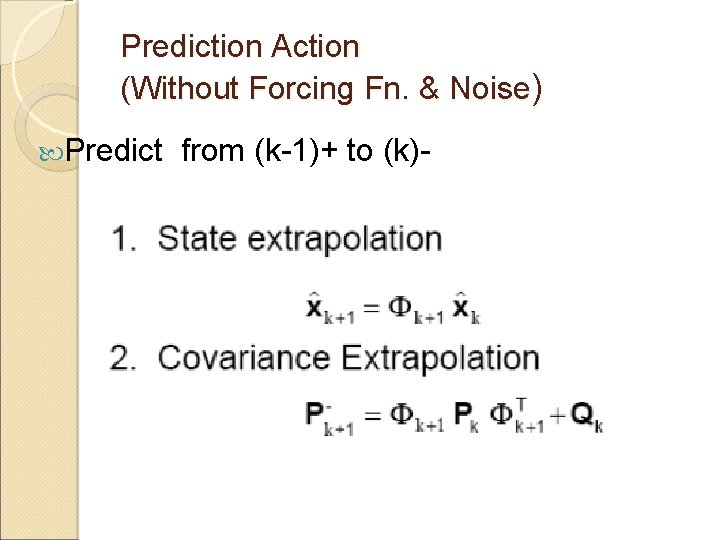Prediction Action (Without Forcing Fn. & Noise) Predict from (k-1)+ to (k)-Update Step Update from (k)- to (k)+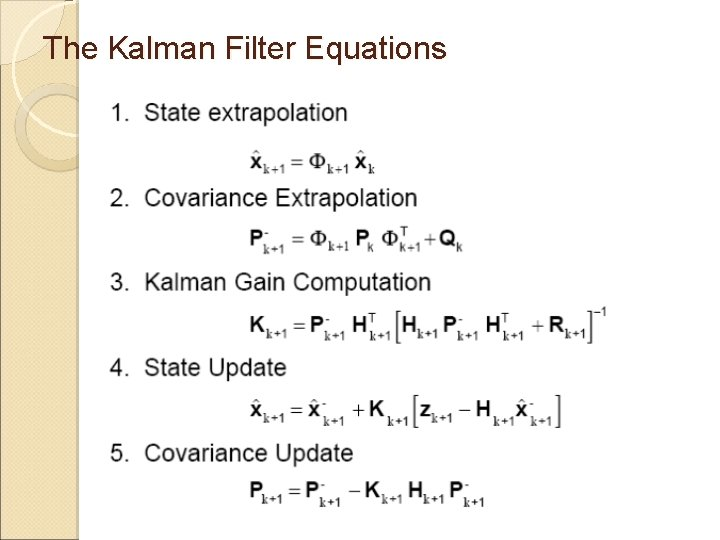The Kalman Filter EquationsNow ! how about the Non-Linear System with Non-Gaussian Uncertainty ? Re-cap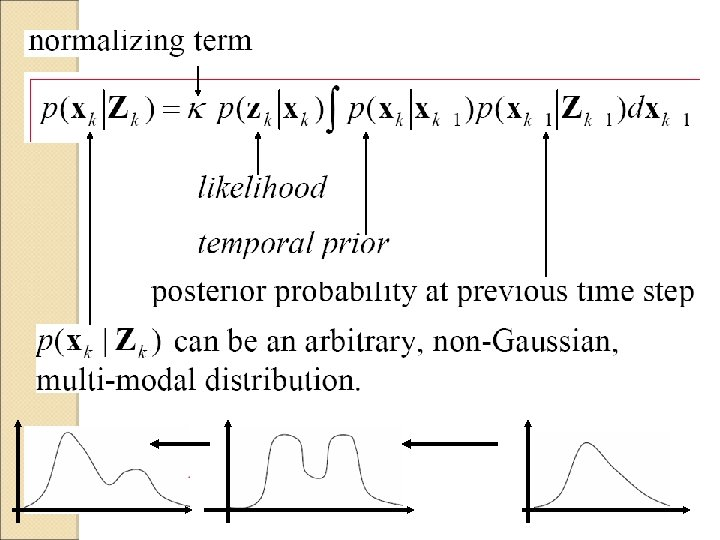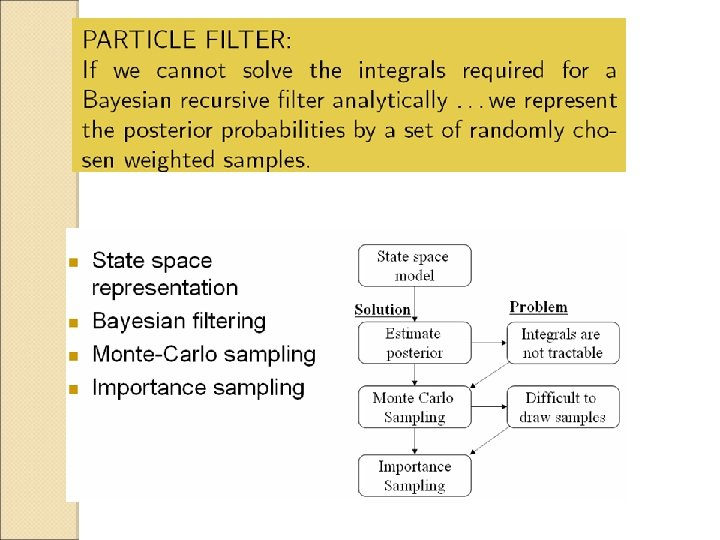Sequential Importance Re-sampling/Particle Filter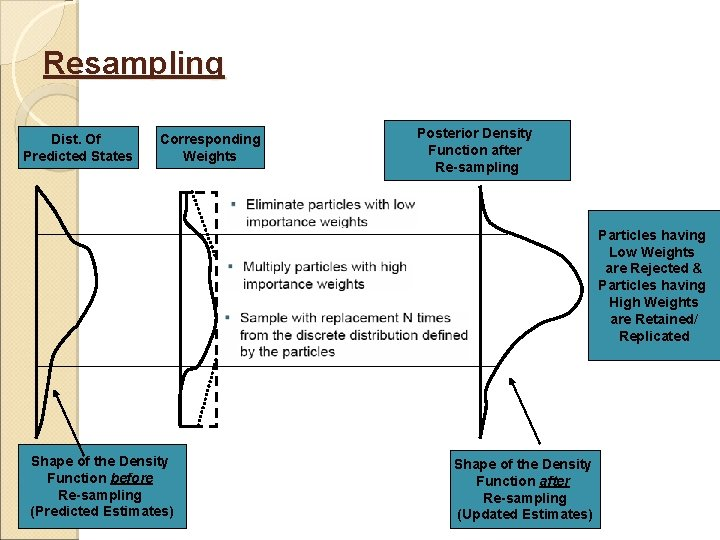Resampling Dist. Of Predicted States Corresponding Weights Posterior Density Function after Re-sampling Particles having Low Weights are Rejected & Particles having High Weights are Retained/ Replicated Shape of the Density Function before Re-sampling (Predicted Estimates) Shape of the Density Function after Re-sampling (Updated Estimates)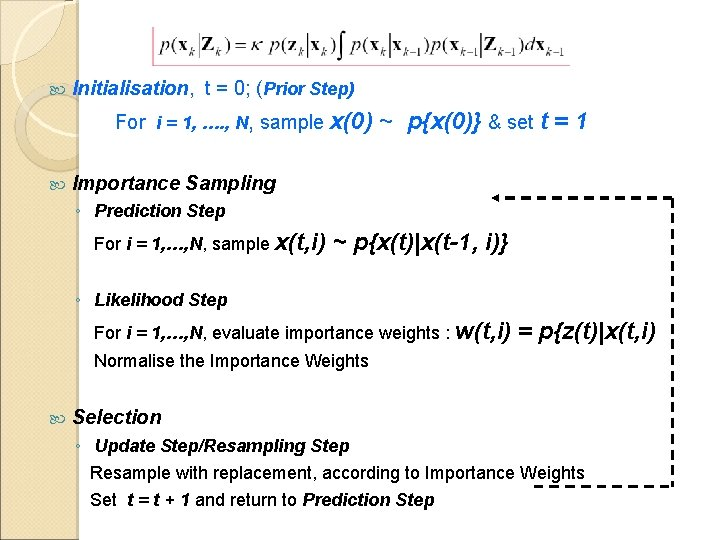Initialisation, t = 0; (Prior Step) For i = 1, …. , N, sample x(0) ~ p{x(0)} & set t = 1 Importance Sampling ◦ Prediction Step For i = 1, …, N, sample x(t, i) ~ p{x(t)|x(t-1, i)} ◦ Likelihood Step For i = 1, …, N, evaluate importance weights : w(t, i) = p{z(t)|x(t, i) Normalise the Importance Weights Selection ◦ Update Step/Resampling Step Resample with replacement, according to Importance Weights Set t = t + 1 and return to Prediction StepPrior For a state vector of dimension (1 x 1) x(: , 1) = sqrt(P 0) * randn(N, 1) We obtain N samples (particles) For a state vector of dimension (n x 1) L = cholesky (P 0) x = randn(N, n) * L + ones(N, n) * x (n x 1) We obtain x of order (N x n)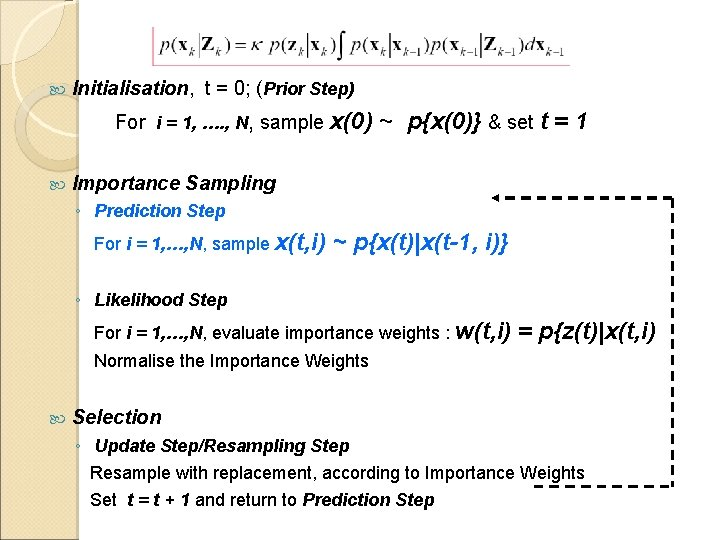Initialisation, t = 0; (Prior Step) For i = 1, …. , N, sample x(0) ~ p{x(0)} & set t = 1 Importance Sampling ◦ Prediction Step For i = 1, …, N, sample x(t, i) ~ p{x(t)|x(t-1, i)} ◦ Likelihood Step For i = 1, …, N, evaluate importance weights : w(t, i) = p{z(t)|x(t, i) Normalise the Importance Weights Selection ◦ Update Step/Resampling Step Resample with replacement, according to Importance Weights Set t = t + 1 and return to Prediction StepPrediction For a state vector of dimension (1 x 1) w ~ sqrt (Q) * randn(N, 1) x(k-) = fn. { x, u } + w We obtain predicted x as (N x 1) vector k-1 For a state vector of dimension (n x 1) w ~Cholesky (Q) * randn(N x n) For i = 1 to N, x 1(k-) = fn. {x, u} + w(i, 1) : xn(k-) = fn. {x, u} + w(i, n) We obtain predicted x as (N x n) vector k k+1Initialisation, t = 0; (Prior Step) For i = 1, …. , N, sample x(0) ~ p{x(0)} & set t = 1 Importance Sampling ◦ Prediction Step For i = 1, …, N, sample x(t, i) ~ p{x(t)|x(t-1, i)} ◦ Likelihood Step For i = 1, …, N, evaluate importance weights : w(t, i) = p{z(t)|x(t, i) Normalise the Importance Weights Selection ◦ Update Step/Resampling Step Resample with replacement, according to Importance Weights Set t = t + 1 and return to Prediction StepLikelihood/Importance Weights Considering an Exponential pdf v = z* ones(N x 1) – C * x (predicted) (1 x n) (n x N) For i = 1 to N Normalising the Weights For i = 1 to N Wt (i) = q(i) / sum(q)Initialisation, t = 0; (Prior Step) For i = 1, …. , N, sample x(0) ~ p{x(0)} & set t = 1 Importance Sampling ◦ Prediction Step For i = 1, …, N, sample x(t, i) ~ p{x(t)|x(t-1, i)} ◦ Likelihood Step For i = 1, …, N, evaluate importance weights : w(t, i) Normalise the Importance Weights = p{z(t)|x(t, i) Selection ◦ Update Step/Resampling Step Resample with replacement, according to Importance Weights Set t = t + 1 and return to Prediction StepResampling Dist. Of Predicted States Corresponding Weights Posterior Density Function after Re-sampling Particles having Low Weights are Rejected & Particles having High Weights are Retained/ Replicated Shape of the Density Function before Re-sampling (Predicted Estimates) Shape of the Density Function after Re-sampling (Updated Estimates)Observations, Y Weights, wi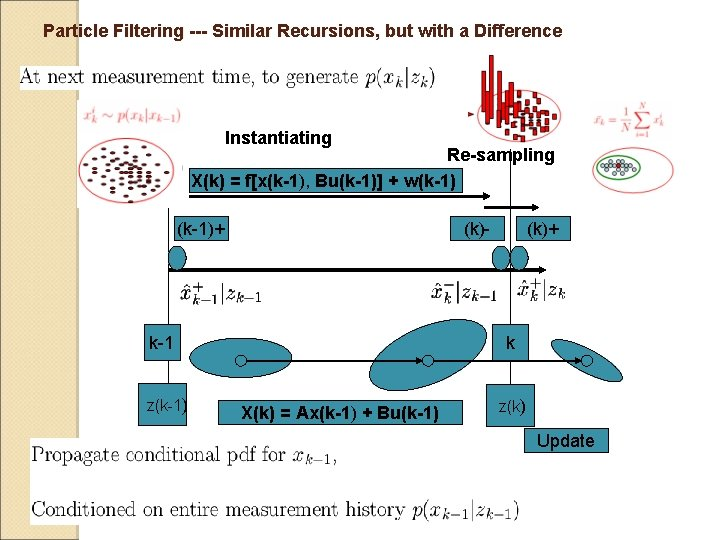Particle Filtering --- Similar Recursions, but with a Difference Instantiating Re-sampling X(k) = f[x(k-1), Bu(k-1)] + w(k-1)+ (k)- k-1 z(k-1) (k)+ k X(k) = Ax(k-1) + Bu(k-1) z(k) Update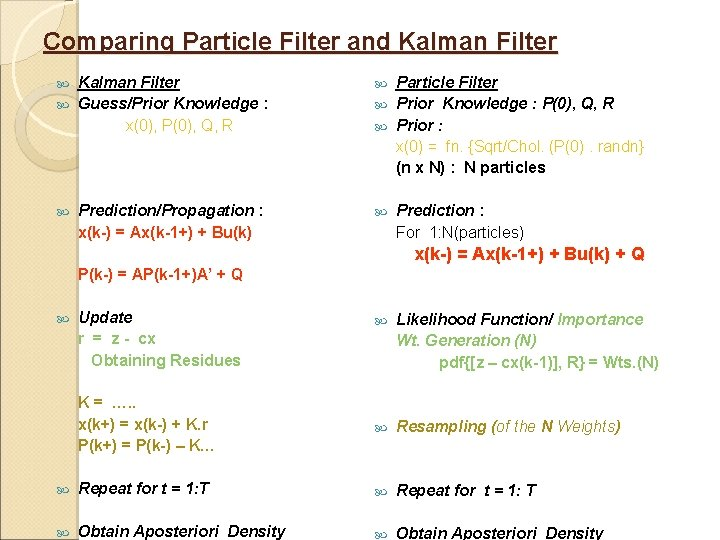Comparing Particle Filter and Kalman Filter Guess/Prior Knowledge : x(0), P(0), Q, R Prediction/Propagation : x(k-) = Ax(k-1+) + Bu(k) Particle Filter Prior Knowledge : P(0), Q, R Prior : x(0) = fn. {Sqrt/Chol. (P(0). randn} (n x N) : N particles Prediction : For 1: N(particles) x(k-) = Ax(k-1+) + Bu(k) + Q P(k-) = AP(k-1+)A’ + Q Update r = z - cx Obtaining Residues Likelihood Function/ Importance Wt. Generation (N) pdf{[z – cx(k-1)], R} = Wts. (N) K = …. . x(k+) = x(k-) + K. r P(k+) = P(k-) – K… Resampling (of the N Weights) Repeat for t = 1: T Obtain Aposteriori Density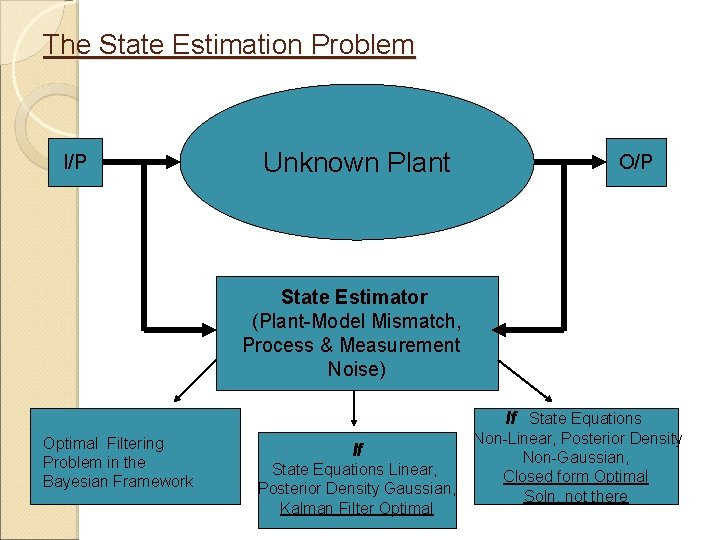The State Estimation Problem I/P Unknown Plant O/P State Estimator (Plant-Model Mismatch, Process & Measurement Noise) If State Equations Optimal Filtering Problem in the Bayesian Framework If State Equations Linear, Posterior Density Gaussian, Kalman Filter Optimal Non-Linear, Posterior Density Non-Gaussian, Closed form Optimal Soln. not there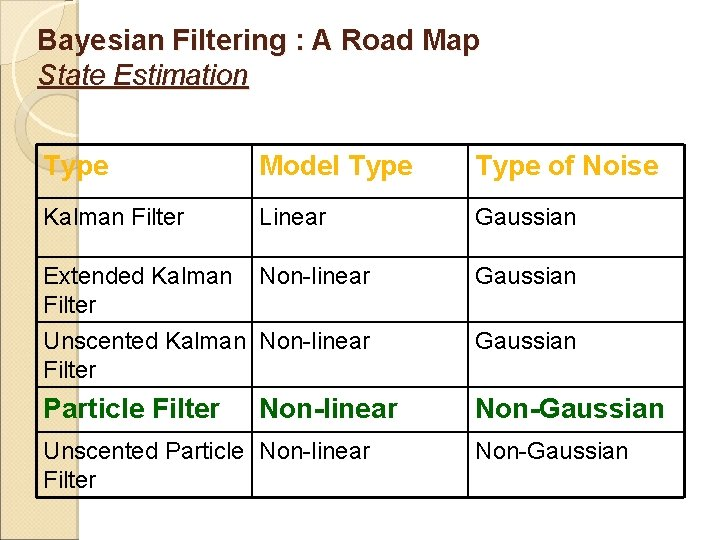Bayesian Filtering : A Road Map State Estimation Type Model Type of Noise Kalman Filter Linear Gaussian Extended Kalman Filter Non-linear Gaussian Unscented Kalman Non-linear Filter Gaussian Particle Filter Non-Gaussian Non-linear Unscented Particle Non-linear Filter Non-Gaussian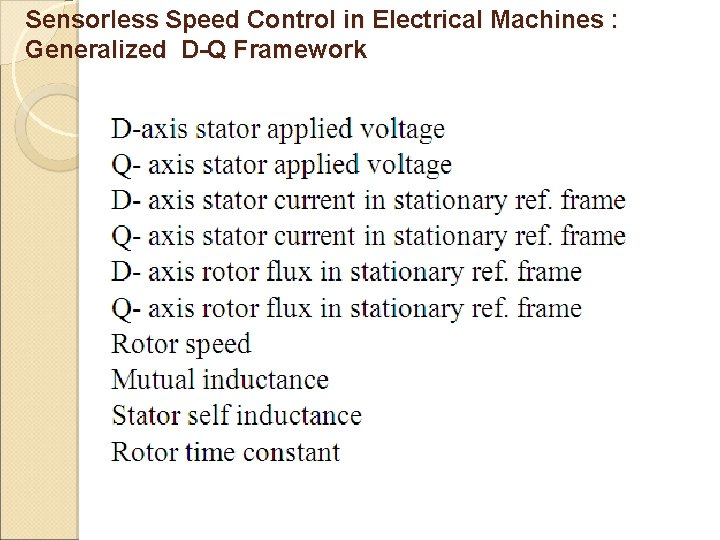Sensorless Speed Control in Electrical Machines : Generalized D-Q FrameworkCasting the D-Q Model in State Space Framework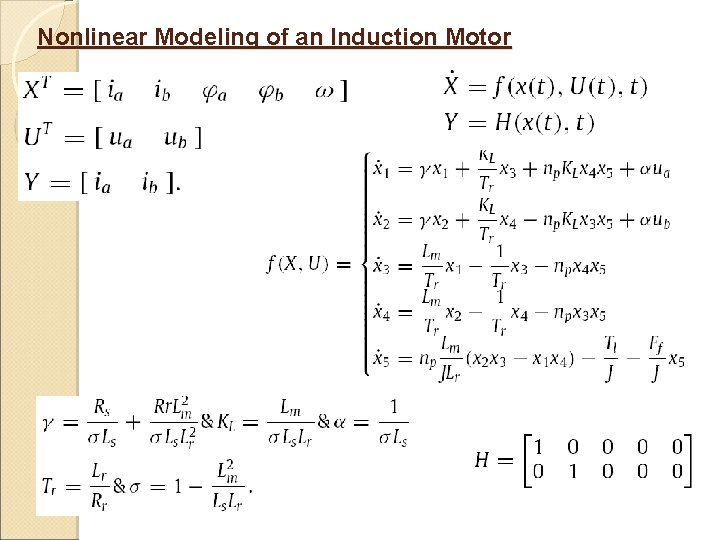Nonlinear Modeling of an Induction MotorEnergy Balance Equation : For Chilled water control Volumes For LCW control Volumes Modelling of the Plate type Heat exchanger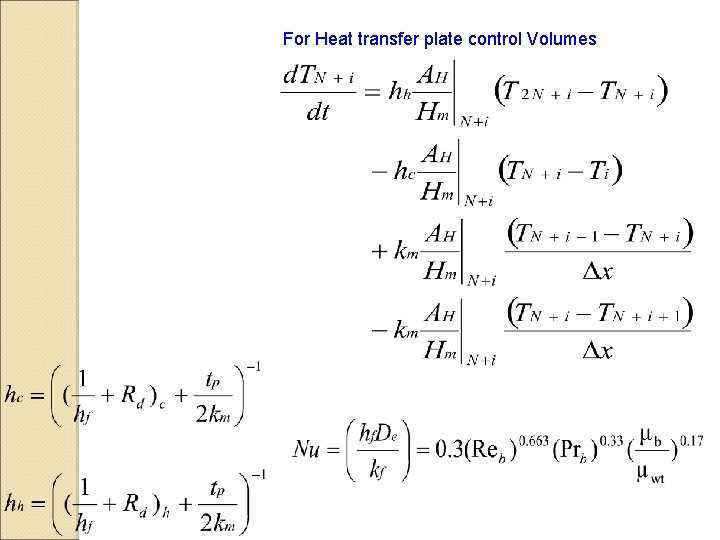For Heat transfer plate control VolumesThe State Space Framework of the Plate type HEX X = [T 1 T 2……T 3 N]T U = [ d. Tchin d. Tlin d. Mche d. Mlcwe ]T Y = [d. Tlout] A = Transition matrix (3 N x 3 N) B = Input matrix (3 N x 4) C = Output matrix (1 x 3 N) D= Feed forward matrix(1 x 4) N is given as input parameter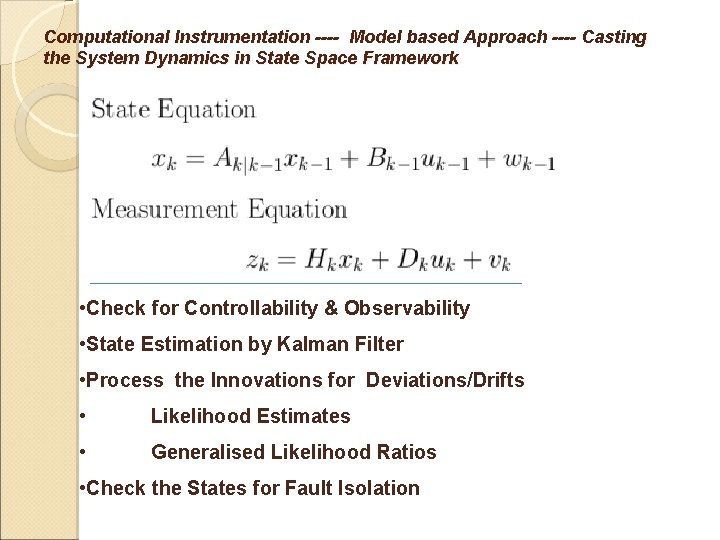Computational Instrumentation ---- Model based Approach ---- Casting the System Dynamics in State Space Framework • Check for Controllability & Observability • State Estimation by Kalman Filter • Process the Innovations for Deviations/Drifts • Likelihood Estimates • Generalised Likelihood Ratios • Check the States for Fault Isolation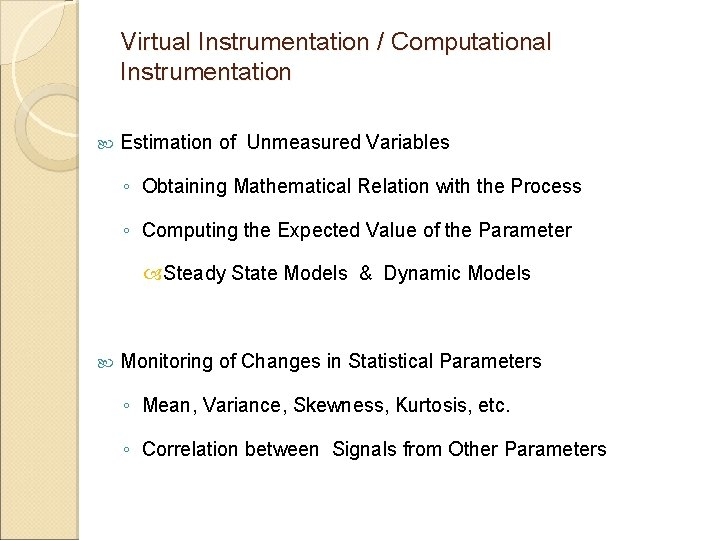Virtual Instrumentation / Computational Instrumentation Estimation of Unmeasured Variables ◦ Obtaining Mathematical Relation with the Process ◦ Computing the Expected Value of the Parameter Steady State Models & Dynamic Models Monitoring of Changes in Statistical Parameters ◦ Mean, Variance, Skewness, Kurtosis, etc. ◦ Correlation between Signals from Other ParametersAvoidance of Common Mode Drifts ◦ Models of Process & Sensor ◦ First-Principles Model (Mechanistic Models) ◦ Empirical Models from Available Data (Data-Based Models) ◦ Estimated Output in Steady State Verification of Calibration over the Entire Operating Range of the Sensor ◦ Piece-wise Comparison with Related Outputs over the Entire Range ◦ Computation from Dynamic Models over the Entire Range Computation of Error Margin between Estimated Output and Actual Output of an Instrument § Calibrate only if there is a Change in the Error Margin, beyond an Acceptable LimitComputational Instrumentation Concepts Use the System Model --- ODE/PDE Check Adequacy of Measurements ◦ No. of States, X ◦ No. of Outputs, Y / Z ◦ Some States may be Measurable To Estimate the Non-Measurable States Recursive Least Square Formulation Bayesian FrameworkThank You For Your Patience